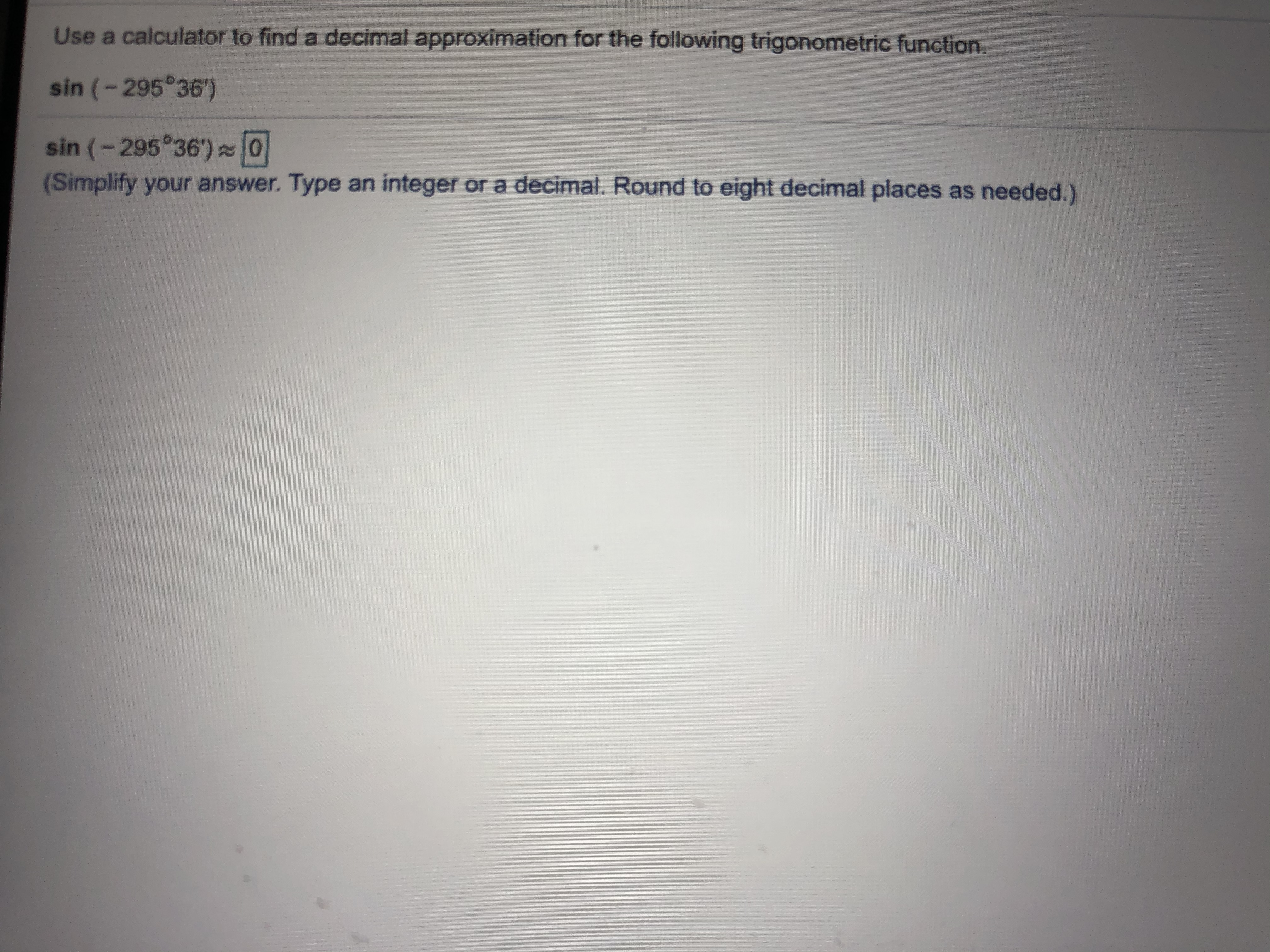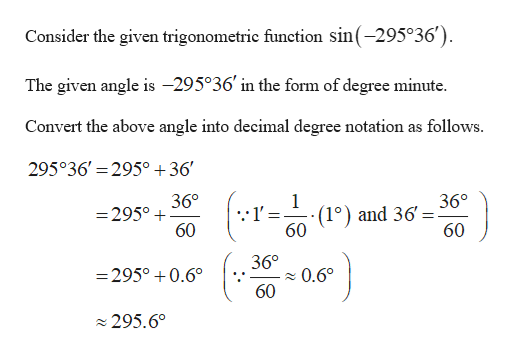Use a calculator to find a decimal approximation for the following trigonometric function.sin (-295°36')sin (-295°36')O(Simplify your answer. Type an integer or a decimal. Round to eight decimal places as needed.)

Questionhelp_outlineImage TranscriptioncloseUse a calculator to find a decimal approximation for the following trigonometric function. sin (-295°36') sin (-295°36')O (Simplify your answer. Type an integer or a decimal. Round to eight decimal places as needed.) fullscreen
Step 1help_outlineImage TranscriptioncloseConsider the given trigonometric function sin(-295°36') The given angle is -295°36 in the form of degree minute Convert the above angle into decimal degree notation as follows. 295°36' 295° +36' 36° 36° (1°) and 36 60 =295° 60 60 36° =295°0.6 0.60 60 295.60 fullscreen

Want to see the full answer?

See Solution

Want to see this answer and more?

Our solutions are written by experts, many with advanced degrees, and available 24/7

See Solution
Tagged in

Trigonometric Ratios